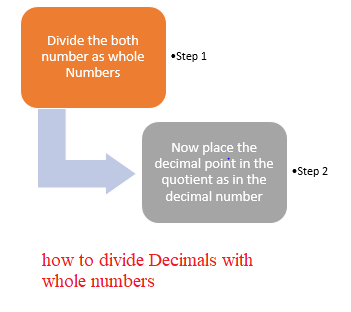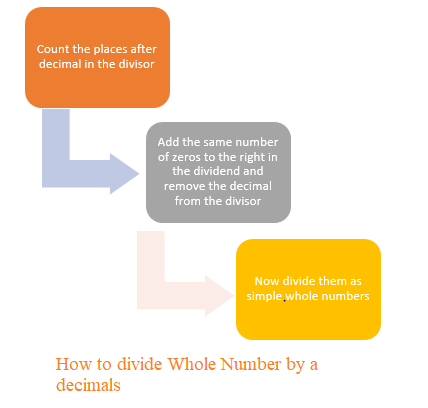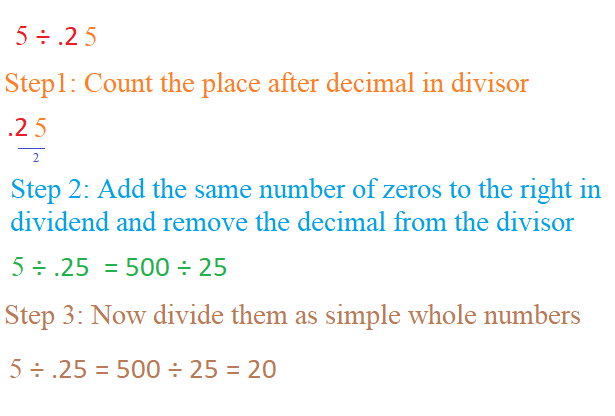# How to Divide decimals | dividing decimals

## Division of Decimals by a Whole Number

how to divide Decimals with whole numbers
Here are the steps to divide Decimals with whole number
a. Divide the both number as whole Numbers
b. Now place the decimal point in the quotient as in the decimal numberLet take the division example
i.$2.8 \div 4$
Step 1: Multiply them as whole number
$28 \div 4 = 7$
Step 2: Now place the decimal point in the quotient as in the decimal number
$.7$

ii. $8.4 \div 4$
Step 1: Multiply the
m as whole number
$84 \div 4 = 21$
Step 2: Now place the decimal point in the quotient as in the decimal number
$2.1$

1. $3.5 \div 7$
$3.5 \div 7= .5$

2. $2.5 \div 5$
$2.5 \div 5=.5$

3. $14.49 \div 7$
$14.49 \div 7= 2.07$

## Division of a Decimals by a 10,100,1000

Division of a Decimals by 10,100,1000 is pretty easy.The digit in the divison remains as same as in the decimal number. Just the decimal point is shifted towards left by the same places as there are zeroes over one

Example i. $423.1 \div 100$.
Step 1: The digit remains same as in the decimals
423.1
Step 2: There are two zeroes over 2
Step 3: Shift the decimal point towards left by 2 places
423.1 becomes 4.231

ii. $.23 \div 1000$.
Step 1: The digit in the product remains as same as in the decimal number
.23
Step 2: There are three zeroes over 1
Step 3: Shift the decimal point towards left by 3 places
.23 becomes .00023

$42.56 \div 100 = .4256$

$76.5 \div 10 = 7.65$
$1455.6 \div 100 = 14.556$
$1.067 \div 10 = 1.067$

## How to divide Whole Number by a decimals

we have already see the division of decimal by whole number.Let us understand How to divide whole number by a decimal.
$4 \div .5= 40 \div 5 = 8$

Here we can see that Whole Number by a decimals is simply acheived by below steps
a. Count the places after decimal in the divisor
b. Add the same number of zeros to the right in the dividend and remove the decimal from the divisor
c. Now divide them as simple whole numbers .Example
i. $2 \div .5$
Step 1: Count the places after decimal in the divisor
Here divisor is .5 and it has 1 place after decimal
Step 2: Add the same number of zeros to the right in the dividend and remove the decimal from the divisor
Here dividend is 2,adding 1 zero ,it becomes 20 and removing decimal fro divisor,divison becomes
$20 \div 5$
Step 3: Now we can divided them as simple whole number
$20 \div 5 =4$1. $11 \div .2$
$11 \div .2 =110 \div 2= 55$

2. $50 \div .2$
$50 \div .2 = 500 \div 2 = 250$

## How to divide decimals by a decimals

Following steps should be done for dividing decimals
a. If the divisor and dividend have same number of places after decimals, we can remove the decimals from them and then just divide then as whole number
b. if the divisor has less number of places after decimals, then First shift the decimal point to the right by equal number of places in both, to convert the divisor to a whole number.Then it becomes division of decimal number by whole number which is already explained above
c. if the divisor has more number of places after decimals,then First shift the decimal point to the right by equal number of places in both, to convert the dividend to a whole number. Then it becomes division of whole number by decimal which is already explained above
Example
i. $2.5 \div .5$
Now both divisor and dividend have same number of places after decimals,so we can remove the decimals from them and then just divide then as whole number
$2.5 \div .5 = 25 \div 5 = 5$

ii. $33.725 \div .25$
Now divisor has less number of places after decimals, then First shift the decimal point to the right by equal number of places in both, to convert the divisor to a whole number.Then it becomes division of decimal number by whole number which is already explained above

$33.725 \div .25 = 3372.5 \div 25 = 134.9$

ii. $2.7 \div .003$
Now the divisor has more number of places after decimals,then First shift the decimal point to the right by equal number of places in both, to convert the dividend to a whole number. Then it becomes division of whole number by decimal which is already explained above
$2.7 \div .003 = 27 \div .03 = 2700 \div 3 = 900$
1. $12.3 \div 4.1$
$12.3 \div 4.1=123 \div 41= 3$

2. $6.25 \div .25$
$6.25 \div .25 = 625 \div 25 = 25$
2. $6.25 \div .25$
$5.5 \div .25 = 55 \div 2.5 =550 \div 25 =22$

### Quiz Time

Question 1$1.21 \div 1.1$?
A) $11$
B)$1.1$
C)$.11$
D)$.01$
Question 2 $3 \div .5$?
A)$60$
B)$.6$
C) $6$
D)None of these
Question 3 $1.44 \div .12$
A)$12$
B)$1.2$
C)$.12$
D)$120$
Question 4 Find the average of 4.2, 3.8 and 7.6
A)$4.3$
B) $4.5$
C) $6$
D) $5.2$
Question 5 A trcuk covers a distance of 89.1 km in 2.2 hours. What is the average distance covered by it in 2 hour??
A)$90$
B)$81$
C)$40.5$
D)$81.5$